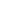# NumPy Fortran-ordering Quiz

Author:
Stéfan van der Walt
http://mentat.za.net

The scoring is simple: four options are given for each question. For a wrong answer a point is deducted, for a correct answer a point is given and choosing 'don't know' does not influence your score.

In this quiz, the question is always the same: "What would the output (e.g. at the Python prompt) be, given the following expression?"

Of course, you are not allowed to use NumPy or python to obtain the answers.

To get you started, here are the docstrings from numpy.array and numpy.reshape:

```Definition: array(object, dtype=None, copy=1,order=None, subok=0,ndmin=0)
[...]
order - Specify the order of the array.  If order is 'C', then the
array will be in C-contiguous order (last-index varies the
fastest). If order is 'FORTRAN', then the returned array will
be in Fortran-contiguous order (first-index varies the
fastest).  If order is None, then the returned array may be in
either C-, or Fortran-contiguous order or even discontiguous.
Definition: N.reshape(a, newshape, order='C')
Docstring: Change the shape of a to newshape.
Return a new view object if possible otherwise return a copy.

```

array([1,2,3,4,5,6],order='F')array([[1,2,3],[4,5,6]],order='F')array([1,2,3,4,5,6],order='C').reshape((2,3),order='F')array([1,2,3,4,5,6],order='F').reshape((2,3),order='C')array([[1,2,3],[4,5,6]]).reshape((2,3),order='F')array([[1,2,3],[4,5,6]],order='F').reshape((2,3),order='C')array([[1,2,3],[4,5,6]]).reshape((3,2),order='F')array([[1,2,3],[4,5,6]],order='F').reshape((3,2),order='C')